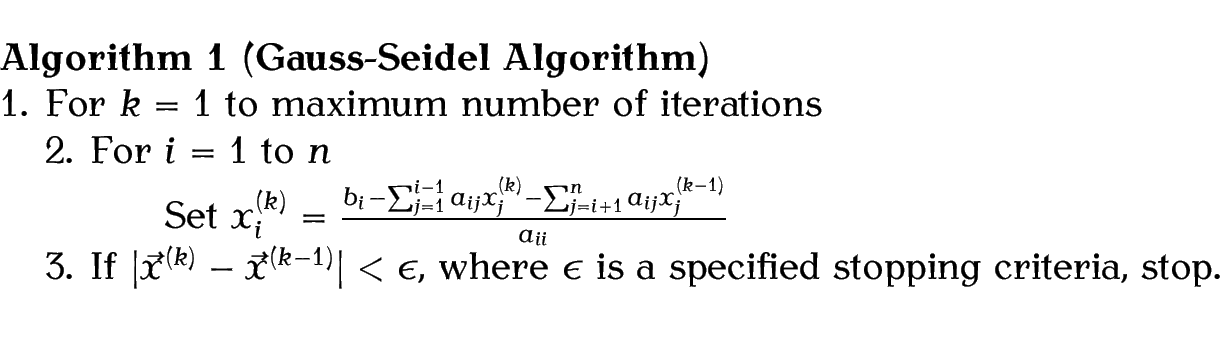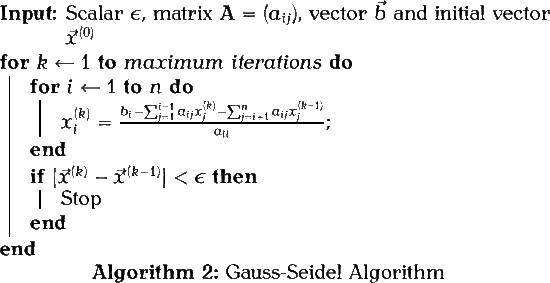# 4.8 Algorithms

If you want to display an algorithm, such as pseudo-code, you can use a combination of the tabbing environment (described in §4.6. Tabbing) and a theorem-like environment (described above in §4.7. Theorems).

Example:

% in the preamble:
\theoremstyle{break}
\theorembodyfont{\normalfont}
\newtheorem{algorithm}{Algorithm}

% later in the document:
\begin{algorithm}[Gauss-Seidel Algorithm]
\begin{tabbing}
1. \=For $k=1$ to maximum number of iterations\\
\>2. For \=$i=1$ to $n$\\
\>\>Set
\begin{math}
x_i^{(k)} =
\frac{b_i-\sum_{j=1}^{i-1}a_{ij}x_j^{(k)}
-\sum_{j=i+1}^{n}a_{ij}x_j^{(k-1)}}
%
{a_{ii}}
\end{math}
\\
\>3. If $\lvert\vec{x}^{(k)}-\vec{x}^{(k-1)}\rvert < \epsilon$,
where $\epsilon$ is a specified stopping criteria, stop.
\end{tabbing}
\end{algorithm}

Result:(See Volume 1 to find out how to redefine \vec to display its argument in bold.)

If you want a more sophisticated approach, there are some packages available on CTAN, such as alg, algorithm2e, algorithms and algorithmicx. I'm going to briefly introduce the algorithm2e package here. This provides the algorithm floating environment. Like the figure and table environments described in Volume 1, the algorithm environment has an optional argument that specifies the placement.

\begin{algorithm}[<placement>]

If you are using a class or package that already defines an algorithm environment, you can use the algo2e package option:

\usepackage[algo2e]{algorithm2e}

This will define an environment called algorithm2e instead of algorithm to avoid conflict.

Within the body of the environment, you must mark the end of each line with \; regardless of whether you want a semi-colon to appear. To suppress the default end-of-line semi-colon, use

To switch it back on again, use

There are a variety of commands that may be used within the algorithm environment. Some of the commands are described below, but for a complete list you should consult the algorithm2e documentation .

First there are the commands for the algorithm input, output and data:

\KwIn{<input>}
\KwOut{<output>}
\KwData{<input>}
\KwResult{<output>}

Next there are commands for basic keywords:

\KwTo
\KwRet{<value>}
\Return{<value>}

There are a lot of conditionals, but here's a selection:

\If{<condition>}{<then block>}
\uIf{<condition>}{<then block without end>}
\ElseIf{<else-if block>}
\uElseIf{<else-if block without end>}
\Else{<else block>}

Similarly there are a lot of loops, but here's a selection:

\For{<condition>}{<body>}
\While{<condition>}{<body>}

Example:

The above algorithm can be written using the algorithm2e environment as follows (this document has used the algo2e package option):

\begin{algorithm2e}
\caption{Gauss-Seidel Algorithm}\label{alg:gauss-seidel}
\KwIn
{%
scalar $\epsilon$,
matrix $\mathbf{A} = (a_{ij})$,
vector $\vec{b}$
and initial vector $\vec{x}^{(0)}$
}

\For{$k\leftarrow 1$ \KwTo maximum iterations}
{
\For{$i\leftarrow 1$ \KwTo $n$}
{
$x_i^{(k)} = \frac { b_i-\sum_{j=1}^{i-1}a_{ij}x_j^{(k)} -\sum_{j=i+1}^{n}a_{ij}x_j^{(k-1)} } % {a_{ii}}$\;
}

\If{$\lvert\vec{x}^{(k)}-\vec{x}^{(k-1)}\rvert < \epsilon$}
{Stop}
}

\end{algorithm2e}

The result is shown in Algorithm 2.The algorithm environment (as defined by algorithm2e without the algo2e option) or algorithm2e environment (as defined with the algo2e option) uses the algocf counter. So in this document, to ensure that the algorithm environment defined with \newtheorem used the same counter as algorithm2e, I had the following in my preamble:

This book is also available as A4 PDF or 12.8cm x 9.6cm PDF or paperback (ISBN 978-1-909440-02-9).

© 2013 Dickimaw Books. "Dickimaw", "Dickimaw Books" and the Dickimaw parrot logo are trademarks. The Dickimaw parrot was painted by Magdalene Pritchett.